# Star and Delta Connection – Explained

Star and Delta Connection – Explained 1024 576 Gaurav J

Star/wye connection and delta connection are the two different methods which are used for connecting 3 phase system. In this video, we’ll learn following in detail.
1. Star Connection
2. Delta connection
3. Voltage and current relationship in both the connections
4. And where these connections are used.
So, if you want to get the details, you need watch the video.

#### Recommend reading before moving on in this tutorial

1 phase and 3 phase power

## Star Connection

Connection shown in the above figure is one of the way to connect 3 phase generator to the load. As you can see this connection requires 6 wires to connect. The three-single phase circuit shown in this figure are electrically independent. But if you observe the image carefully you’ll find that, we can combine the three return conductors together to form a single return conductor. As a result, we have saved cost of two conductors, as the number of conductor are reduced from 6 to 4.

Now, this common return conductor is called as Neutral conductor. It carries sum of three currents Ia + Ib + Ic. At first, many of the people will think that, the conductor required for neutral should be 3 times in the size than the other three conductors. But, as we know, current of the three phases are out of phase by 120 deg from each other. And if we draw a waveform for these current it will look like this.

Now, if you observe the diagram carefully, you’ll find that the sum of return currents is zero at every instance. For example, at instant shown above, corresponding to 240 deg, Ic = Imax and Ib = Ia = -0.5 Imax.
So, if we add these return currents, we’ll get the sum =0, and this is true for every instance.

Ia + Ib + Ic = (-0.5Imax – 0.5Imax) + Imax
Ia + Ib + Ic = – Imax + Imax = 0

We can therefore, remove the neutral conductor without affecting the voltage or current in the circuit. So, we have reduced the number of conductors 6 which was the initial stage to 3. 50% saving in conductor cost! However, to achieve this, the load shown in the circuit must be identical. If the load is not identical, then removing neutral conductor can cause unequal voltages across the loads. So, in an ideal situation or in the situation where load is equal, current flowing through neutral conductor is Zero. And this is also a very commonly used question in technical interviews. Does neutral carries current or not? And, you have the answer now.

The conditions where load is not equal, a neutral must be provided. You might have heard people saying 3.5 core cable, that 0.5 core is your neutral conductor. The circuit shown in the figure is called as 3 phase 3 wire system. The generator and the load are said to be connected in wye, as it resembles the letter Y or some people also called it as STAR.

The figure shown above is called as 3 phase 4 wire system. The neutral conductor can be of similar size or may be slightly smaller than the other conductors. 3 phase 4 wire system is widely used to supply power to commercial and industrial customers.

### Voltage & current in Start connection

Now, when we talk about star connected three phase system, we must know about these two concepts.

1. Line to line voltage
2. Line to neutral voltage.

The voltage between A and N is called as line to neutral voltage. Similarly, voltage between A and B is called as line to line voltage. Relation between these voltages as well as current changes with the type of connection. So, it is important to understand these relationships for different connections.

#### Current is Star connected system

In case of star connection, line to neutral current is equal to line to line current.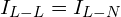#### Voltage in Star connected system

But in case of voltage, it is different. Consider the above star connected 3 phase 4 wire system. If you apply the kirchoff’s voltage law to the above circuit, you’ll find that, line to line voltage is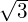times the line to neutral voltage.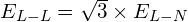Voltage which we receive in our home is the Line to neutral voltage I.e. 230 volts (in India) .

## Delta Connection

One of the other way to connect three phase system is called as delta connection. The connection is so named because it resembles the greek letter delta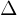.

### Voltage & current in Delta connection

Let have a look at the voltage and currents relationship in delta connection.

#### Voltage in Delta connection

Now in case of delta connection, voltage across each connection is same as line voltage.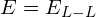#### Current in Delta connection

But, in case of current, current across each element is different than the line current. If you apply the Kirchhoff’s law and do some math, you’ll find that the line current is root 3 times greater than the current in each branch of delta connected system.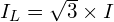## Application of Star & Delta Connection

Generally, star connection is used where you need a neutral and two separate voltages, like our distribution system.
Delta connection is generally preferred where neutral conductor is not needed like for transmission of high voltage power. Also, delta connection is preferred where 3rd harmonics needs to controlled.

Star and delta connections are almost used everywhere when we talk about 3 phase system.

You’ll generally find 3 phase transformer connected in different combinations of star and delta connection. For example,

• Star – star connected transformer is generally used as an auto transformer.
• Delta – delta connected transformer is generally used for high voltage transmission.
• Delta – star connected transformer is generally used as a distribution transformer.

## Power transmitted by star & Delta connections.

Now, as we have studied the star & delta connection, a question can pop up, power transferred by a star connection and power transfer by a delta connection is same or different? So, let’s find that out.

Consider the star connected winding first. Apparent power transferred by a single phase is given by.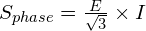This is the power transferred by a single phase. To calculate the power transferred by 3 phase we can multiply this equation by 3.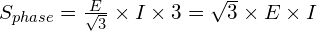Similarly, Apparent power transferred by a single phase of delta connection is given by.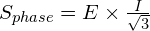Multiply above equation by 3 to calculate power transferred by 3 phase. And you’ll get the same result as star connection.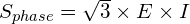And this proves, power transmitted by both the connection is same.

## Summary

So, let’s summarise this tutorial.

1. 3 phase system can be connected in two different styles I.e. star or delta.
2. In star connection, line to line current is equal to line to neutral current. But, line to line voltage is root 3 times the line to neutral voltage.
3. In delta connection, voltage across each element is equal to line voltage. But, line current is root 3 times the current flowing through each element.
4. Power transmitted by both the connection is same.###### Gaurav J

Electrical Engineer. Content Creator. Currently working with a High & Extra High Voltage Switchgear Industry.

All stories by: Gaurav J